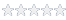# Determining the frequency measurement accuracy of the GTX2200 and GC2200…

#### Knowledge Base Article # Q200094

Summary The GTX2200 family uses a technique known as Reciprocal Counting for measuring frequency. This is a method where the accuracy of the measurement is based on the relationship of the reference clock period and the measurement window duration (gate time), and not the input signal frequency.Login to rate article
For frequency measurements, the GTX2200 family uses a technique known as Reciprocal Counting, a method where the accuracy of the measurement is based on the relationship of the reference clock period and the measurement window duration (gate time), and not the input signal frequency.

This method measures the time interval between two identical trigger points on the input signal, as defined by the trigger level setting and the trigger slope setting.  During the gate time – the window in which the instrument is taking a measurement, the instrument simultaneously counts the number of cycles of the input signal, and the number of clock pulses from the built-in frequency reference.  A microprocessor then calculates the frequency of the input signal.  The absolute resolution of a measurement is the period of one reference clock cycle, and the relative resolution is equal to the absolute resolution divided by the gate time.

For example, if the counter incorporates a 100MHz reference clock, then the absolute resolution is eight digits (10nS = 1.0E-8 Seconds).  Using a gate time of 100mS, the relative resolution is seven digits irrespective of the input signal frequency (10 nS/0.1S = 1.0E-7 Seconds).  The GTX2210, GTX2220 and GTX2230 all use a 100 MHz reference clock, but the GTX2220 and GTX2230 use an interpolation technique which yields an effective absolute resolution of 10 digits, equivalent to a 10 GHz reference clock.

The overall accuracy of the measurement is degraded by the combined effects of the jitter on the measured signal, the jitter on the reference clock, the accuracy of trigger detection circuit and the accuracy of the gate timer.  The frequency measurement accuracies for the GTX2200 family of products are:

GTX2210/GC2210:
#DigitsGate Time (s)
81.000
70.100
60.010
50.001

GTX2220/GC2220 and GTX2230/GC2230:
#DigitsGate Time (s)
101.000
90.100
80.010
70.001

Article Date 6/17/2008
Keywords GTX2200, GC2200, Counter

 Login to rate article>